Question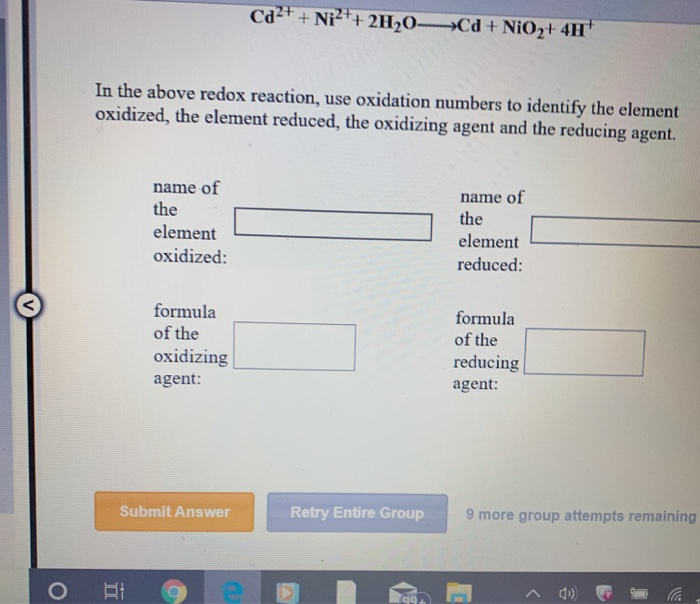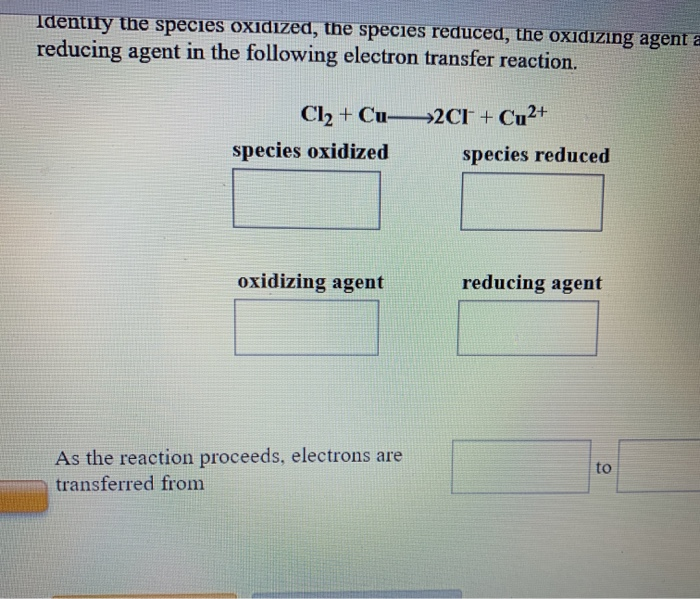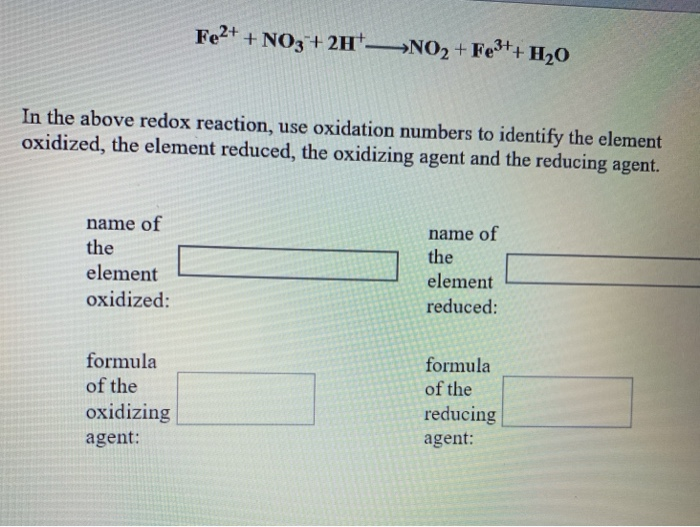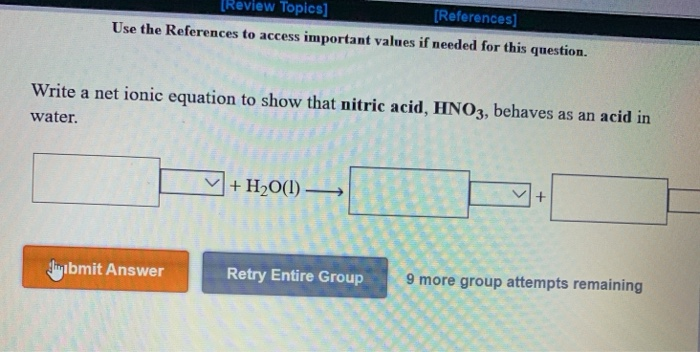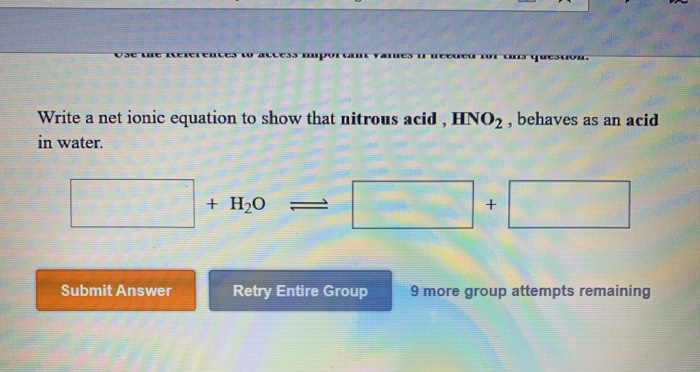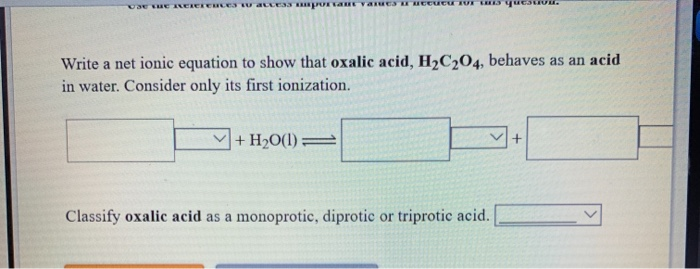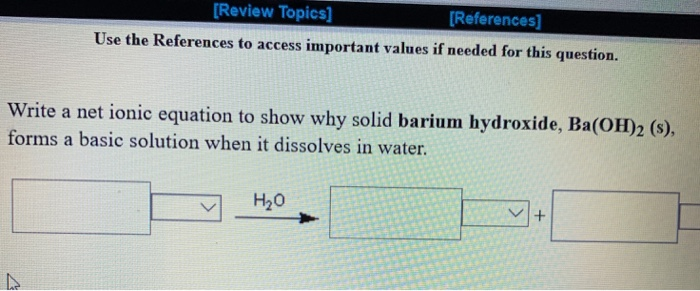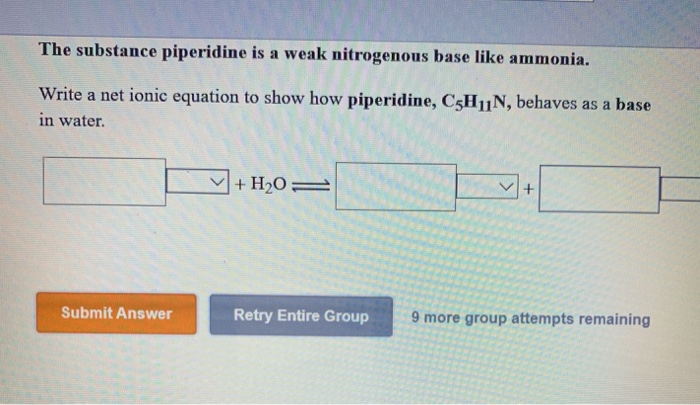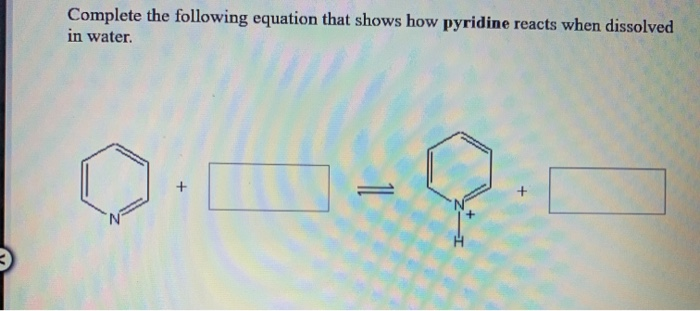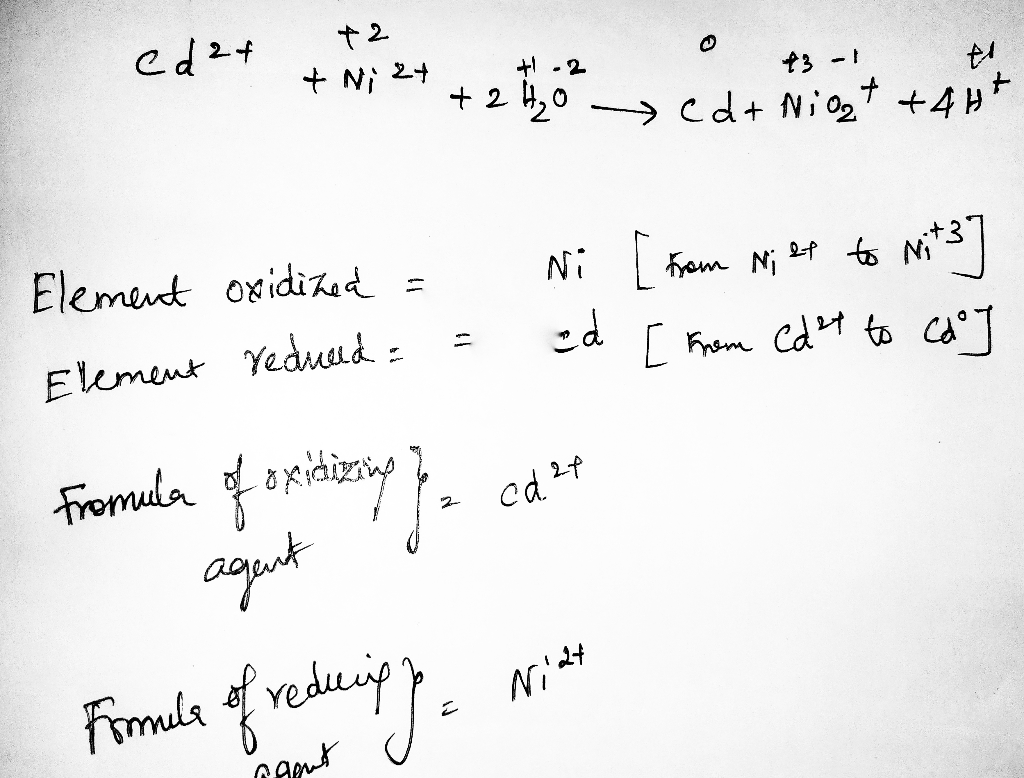#### Earn Coins

Coins can be redeemed for fabulous gifts.

Similar Homework Help Questions
• ### Use the References to access important values if needed for this question. Identify the species oxidized, the sp...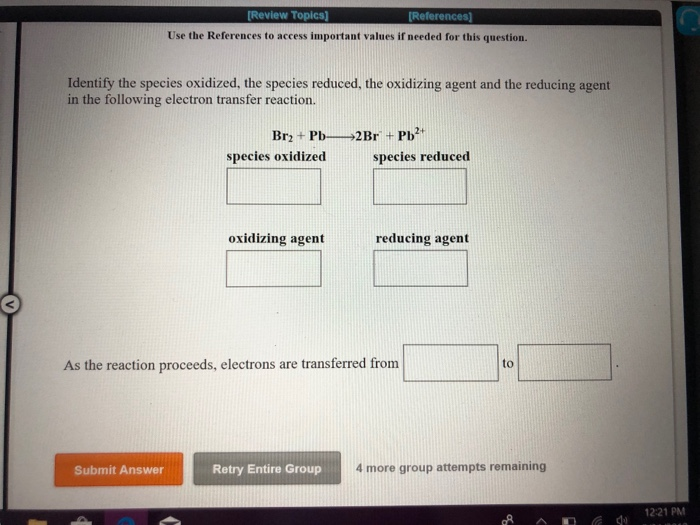Use the References to access important values if needed for this question. Identify the species oxidized, the species reduced, the oxidizing agent and the reducing agent in the following electron transfer reaction. species oxidized species reduced oxidizing agent reducing agent As the reaction proceeds, electrons are transferred from to Retry Entire Group 4more group attempts remaining Submit Answer 1221 PM References Use the References to access important values if needed for this question. Identify the species oxidized, the species reduced,...

• ### Use the References to access impertant values if needed for this question In the above redox...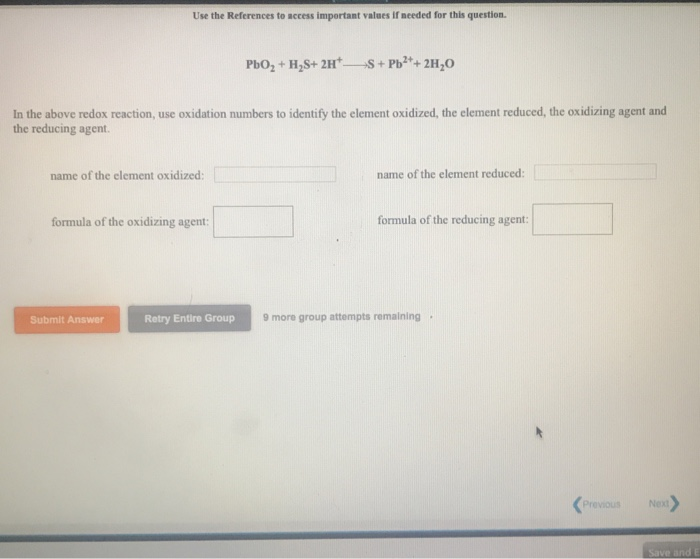Use the References to access impertant values if needed for this question In the above redox reaction, use oxidation numbers to identify the element oxidized, the element reduced, the oxidizing agent and the reducing agent. name of the element oxidized: name of the element reduced: formula of the oxidizing agent! ] formula of the reducing agent Submit Answer Retry Entire Group 9 mo group attempts remaining . Previous Ne

• ### Ni + PbO2+ 4H+ Pb2+ + Ni2++ 21,0 In the above redox reaction, use oxidation numbers...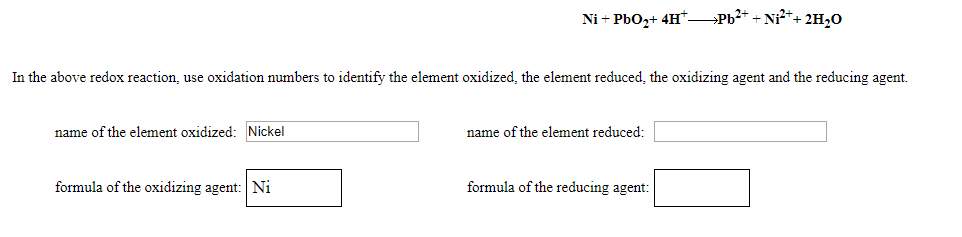Ni + PbO2+ 4H+ Pb2+ + Ni2++ 21,0 In the above redox reaction, use oxidation numbers to identify the element oxidized the element reduced the oxidizing agent and the reducing agent. name of the element oxidized: Nickel name of the element reduced: formula of the oxidizing agent: Ni formula of the reducing agent:

• ### 1. 3NiO2 + 2NO+ 4H+ ------------------------3Ni2+ + 2NO3-+ 2H2O In the above redox reaction, use oxidation...

1. 3NiO2 + 2NO+ 4H+ ------------------------3Ni2+ + 2NO3-+ 2H2O In the above redox reaction, use oxidation numbers to identify the element oxidized, the element reduced, the oxidizing agent and the reducing agent. name of the element oxidized: name of the element reduced: formula of the oxidizing agent: formula of the reducing agent: 2. 2NO2 + Cu2++ 2H2O--------------------------Cu + 2NO3-+ 4H+ In the above redox reaction, use oxidation numbers to identify the element oxidized, the element reduced, the oxidizing agent and...

• ### Ni(OH)2 + NO3- _______> NiO2 + NO2- + H2O In the above redox reaction, use oxidation numbers to identify the...

Ni(OH)2 + NO3- _______> NiO2 + NO2- + H2O In the above redox reaction, use oxidation numbers to identify the element oxidized, the element reduced, the oxidizing agent and the reducing agent. name of the element oxidized:    name of the element reduced: formula of the oxidizing agent: formula of the reducing agent:

• ### 2BrO35NO2 H20 >Br2+ 5NO3+ 20H In the above redox reaction, use oxidation numbers to identify the...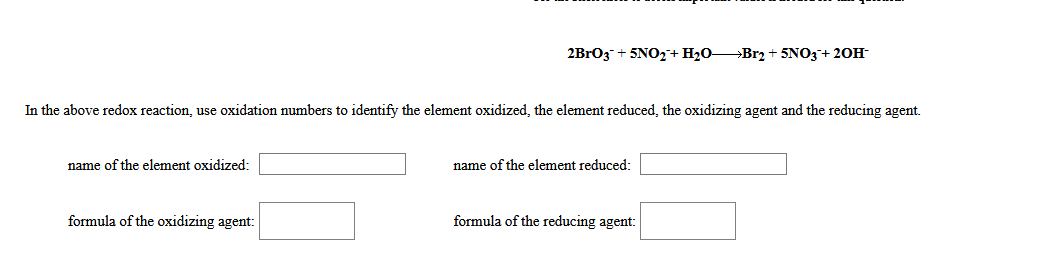2BrO35NO2 H20 >Br2+ 5NO3+ 20H In the above redox reaction, use oxidation numbers to identify the element oxidized, the element reduced, the oxidizing agent and the reducing agent. name of the element oxidized: name of the element reduced: formula of the oxidizing agent formula of the reducing agent

• ### Cu(OH)2 + Hg→→→→HgO + Cu+ H2O In the above redox reaction, use oxidation numbers to identify...

Cu(OH)2 + Hg→→→→HgO + Cu+ H2O In the above redox reaction, use oxidation numbers to identify the element oxidized, the element reduced, the oxidizing agent and the reducing agent. name of the element oxidized: name of the element reduced: formula of the oxidizing agent: formula of the reducing agent: ------------------------------------------------------------------------------------------------------------------------------------------------------------------------------------------------------------------------------------- 3Ag2O + 2Bi+ 3H2O→→→→2Bi(OH)3 + 6Ag In the above redox reaction, use oxidation numbers to identify the element oxidized, the element reduced, the oxidizing agent and the reducing agent. name...

• ### 2NO 3CIO4H202HNO3+ 3CI03 In the above redox reaction, use oxidation numbers to identify the element oxidized,...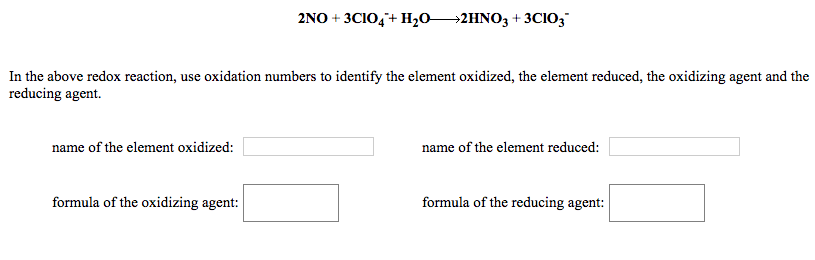2NO 3CIO4H202HNO3+ 3CI03 In the above redox reaction, use oxidation numbers to identify the element oxidized, the element reduced, the oxidizing agent and the reducing agent. name of the element reduced name of the element oxidized: formula of the oxidizing agent formula of the reducing agent:

• ### 6HCIO + 2NO3Cl2 + 2NO3 + 2H+ 2H20 In the above redox reaction, use oxidation numbers to identify the element oxidiz...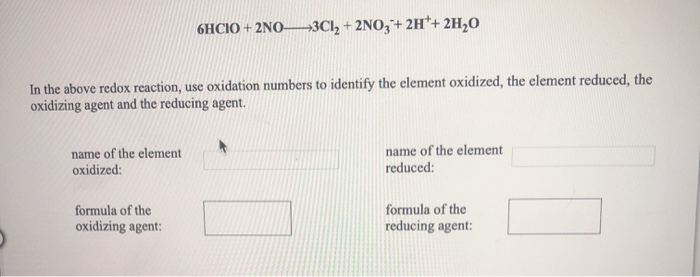6HCIO + 2NO3Cl2 + 2NO3 + 2H+ 2H20 In the above redox reaction, use oxidation numbers to identify the element oxidized, the element reduced, the oxidizing agent and the reducing agent. name of the element oxidized: name of the element reduced: formula of the oxidizing agent: formula of the reducing agent:

• ### 2Cut + 2CO2+ 217—2Cu2+ + H,C204 In the above reaction, the oxidation state of copper changes...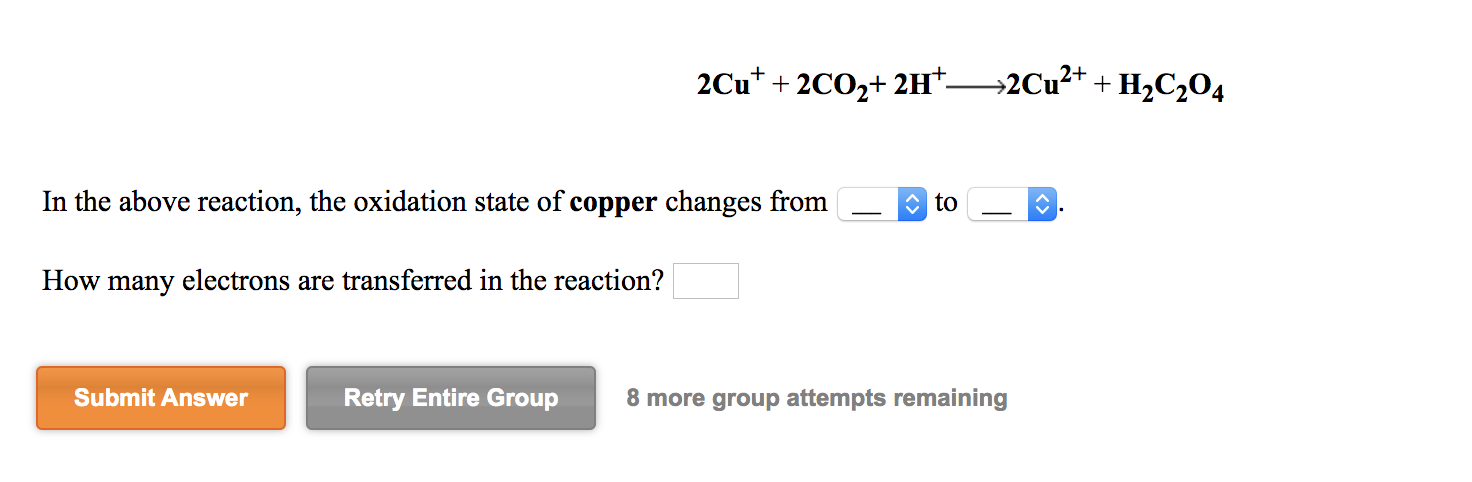2Cut + 2CO2+ 217—2Cu2+ + H,C204 In the above reaction, the oxidation state of copper changes from to - How many electrons are transferred in the reaction? Submit Answer Retry Entire Group 8 more group attempts remaining Identify the species oxidized, the species reduced, the oxidizing agent and the reducing agent in the following electron transfer reaction. Pb2+ + Ni—→ Pb + Ni2+ species oxidized species reduced oxidizing agent reducing agent As the reaction proceeds, electrons are transferred from Submit...